### Discover more content...

Enter some keywords in the search box above, we will do our best to offer you relevant results.

### We're sorry!

We couldn't find any results for your search. Please try again with another keywords.

## 一. Motivations## 二. Neural Networks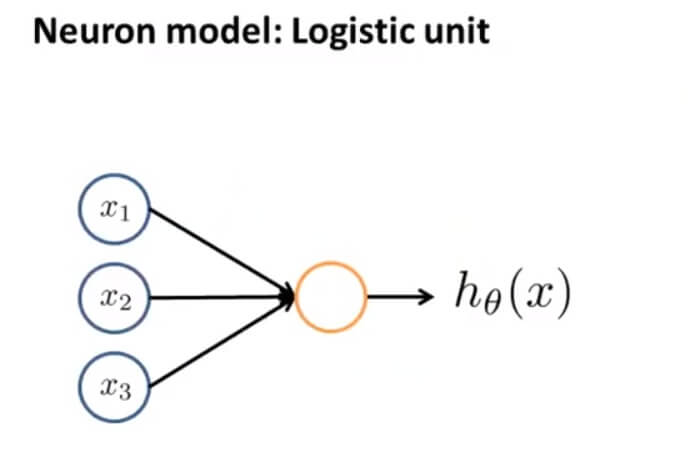$x_{1},x_{2},x_{3}$ 可以将其看成输入神经树突，黄色的圆圈则可以看成中心处理器细胞核， $h_\theta(x)$ 则可看成输出神经轴突。因为这里是逻辑单元，所以我们的输出函数为： $h_\theta(x)=\frac{1}{1+e^{-\theta^Tx}}$ 。一般我们把这称为一个有 s 型函数（逻辑函数）作为激励的人工神经元。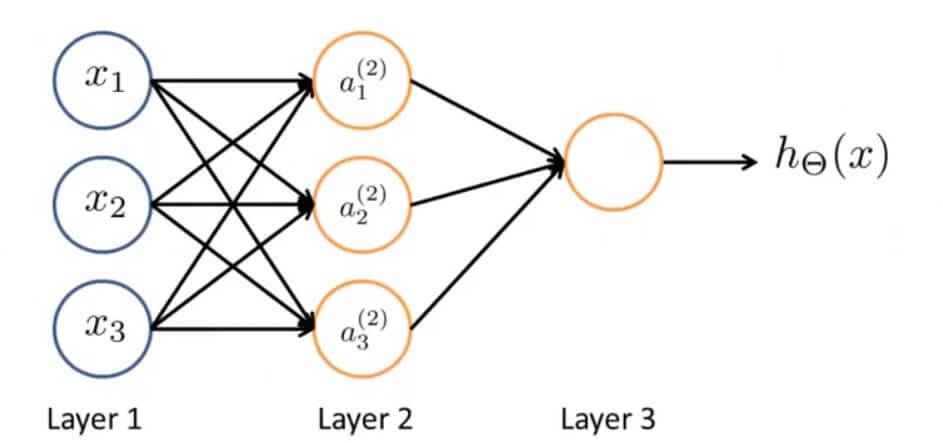• 阶跃函数。这是最简单直接的形式，也是人工神经网络定义时一般采用的。
• 逻辑函数。就是S型函数（Sigmoid函数），具有可无限微分的优势。
• 斜坡函数
• 高斯函数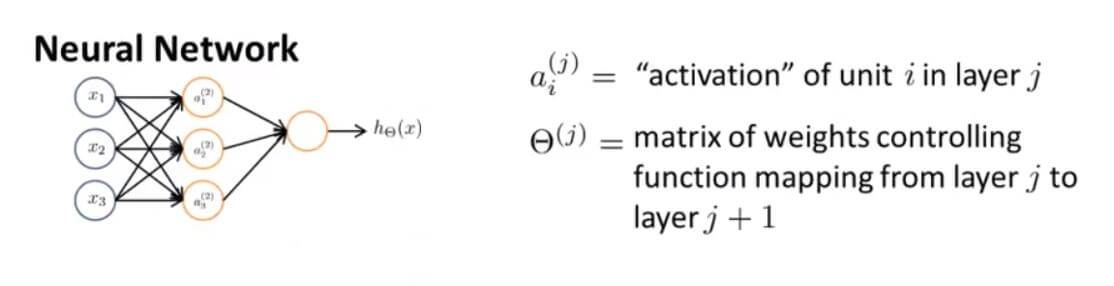$$\begin{bmatrix} x_{0}\\ x_{1}\\ x_{2}\\ x_{3} \end{bmatrix} \rightarrow \begin{bmatrix} a_{1}^{(2)}\\ a_{2}^{(2)}\\ a_{3}^{(2)} \end{bmatrix} \rightarrow h_{\theta}(x)$$

\begin{align*} a_{1}^{(2)} &= g(\Theta_{10}^{(1)}x_{0}+\Theta_{11}^{(1)}x_{1}+\Theta_{12}^{(1)}x_{2}+\Theta_{13}^{(1)}x_{3}) \\ a_{2}^{(2)} &= g(\Theta_{20}^{(1)}x_{0}+\Theta_{21}^{(1)}x_{1}+\Theta_{22}^{(1)}x_{2}+\Theta_{23}^{(1)}x_{3}) \\ a_{3}^{(2)} &= g(\Theta_{30}^{(1)}x_{0}+\Theta_{31}^{(1)}x_{1}+\Theta_{32}^{(1)}x_{2}+\Theta_{33}^{(1)}x_{3}) \\ h_{\Theta}(x) &= a_{1}^{(3)} = g(\Theta_{10}^{(2)}a_{0}^{(2)}+\Theta_{11}^{(2)}a_{1}^{(2)}+\Theta_{12}^{(2)}a_{2}^{(2)}+\Theta_{13}^{(2)}a_{3}^{(2)}) \\ \end{align*}

$\Theta$ 矩阵也被称作为模型的权重。这里的 $g(x)$ 都是 sigmoid 激活函数，即 $g(x) = \frac{1}{1+e^{-x}}$

\begin{align*} z_{1}^{(2)} &= \Theta_{10}^{(1)}x_{0}+\Theta_{11}^{(1)}x_{1}+\Theta_{12}^{(1)}x_{2}+\Theta_{13}^{(1)}x_{3} \\ z_{2}^{(2)} &= \Theta_{20}^{(1)}x_{0}+\Theta_{21}^{(1)}x_{1}+\Theta_{22}^{(1)}x_{2}+\Theta_{23}^{(1)}x_{3} \\ z_{3}^{(2)} &= \Theta_{30}^{(1)}x_{0}+\Theta_{31}^{(1)}x_{1}+\Theta_{32}^{(1)}x_{2}+\Theta_{33}^{(1)}x_{3} \\ \vdots \\ z_{k}^{(2)} &= \Theta_{k,0}^{(1)}x_{0}+\Theta_{k,1}^{(1)}x_{1}+\Theta_{k,2}^{(1)}x_{2}+\Theta_{k,3}^{(1)}x_{3} \\ \end{align*}

\begin{align*} a_{1}^{(2)} &= g(z_{1}^{(2)}) \\ a_{2}^{(2)} &= g(z_{2}^{(2)}) \\ a_{3}^{(2)} &= g(z_{3}^{(2)}) \\ \end{align*}

$$x = \begin{bmatrix} x_{0}\\ x_{1}\\ x_{2}\\ x_{3} \end{bmatrix},z^{(2)} = \begin{bmatrix} z_{1}^{(2)}\\ z_{2}^{(2)}\\ z_{3}^{(2)}\\ \end{bmatrix} = \Theta^{(1)}x$$

\begin{align*} x &= \begin{bmatrix} x_{0}\\ x_{1}\\ \vdots \\ x_{n} \end{bmatrix},z^{(j)} = \begin{bmatrix} z_{1}^{(j)}\\ z_{2}^{(j)}\\ \vdots \\ z_{3}^{(j)}\\ \end{bmatrix}, \\ \Rightarrow z^{(j)} &=\Theta^{(j-1)}a^{(j-1)}\\ \end{align*}

\begin{align*} a^{(j)}&=g(z^{(j)})\\ z^{(j+1)}&=\Theta^{(j)}a^{(j)}\\ h_\Theta(x)&=a^{(j+1)}=g(z^{(j+1)})\\ \end{align*}

## 三. Applications

### 1. 逻辑运算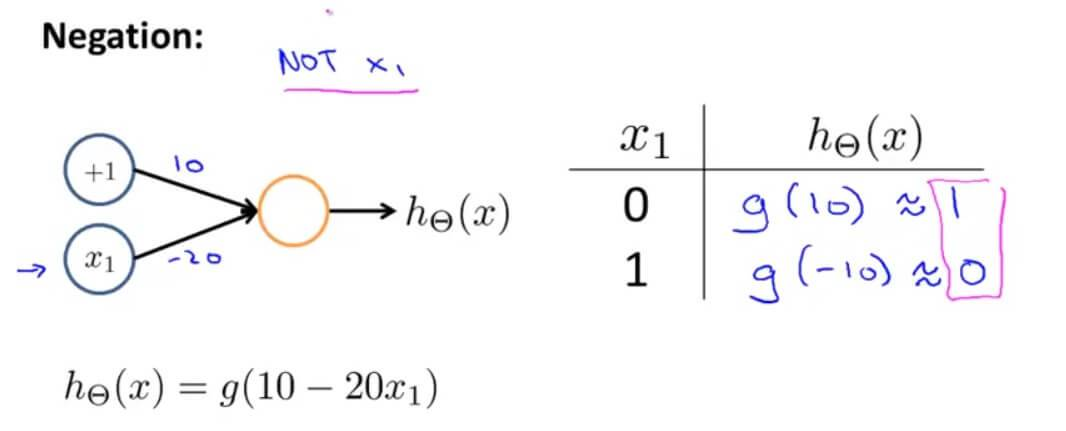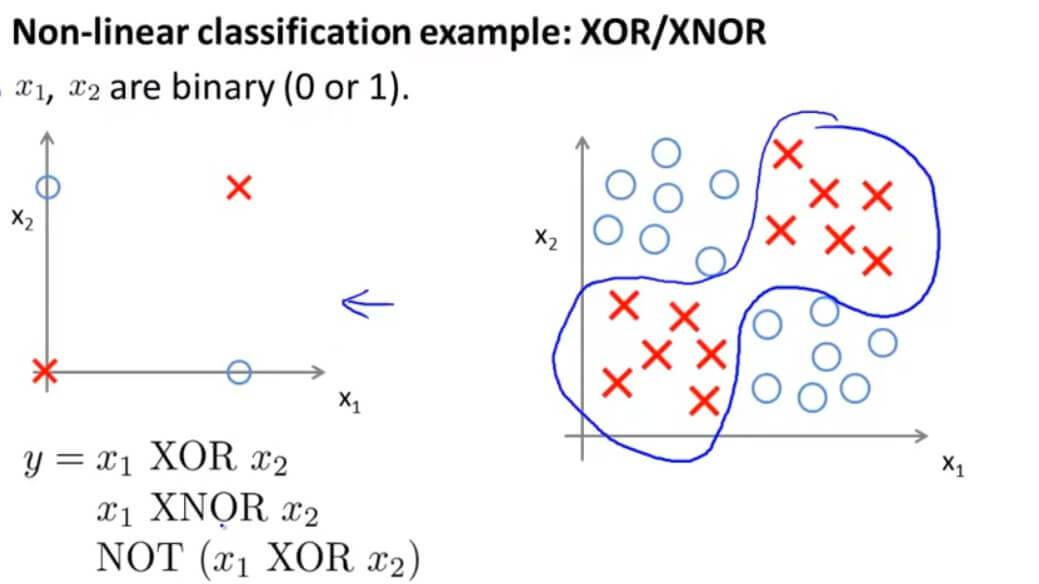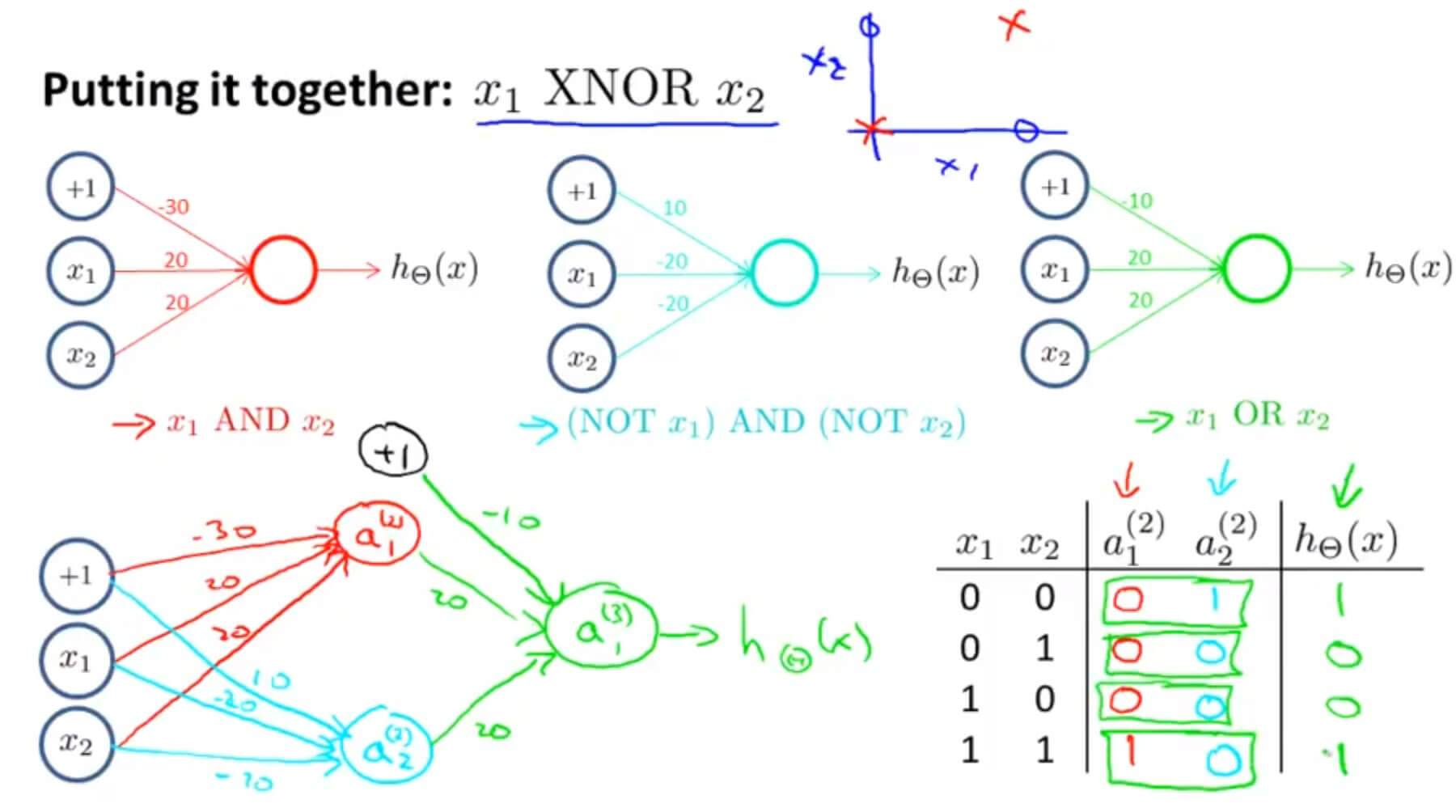### 2. 本质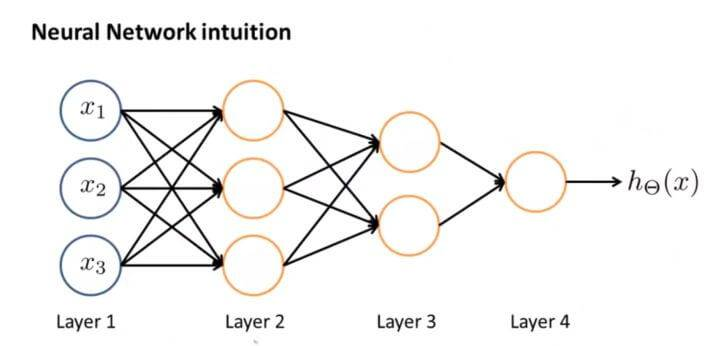## 四. Neural Networks: Representation 测试

### 1. Question 1

Which of the following statements are true? Check all that apply.

A. Suppose you have a multi-class classification problem with three classes, trained with a 3 layer network. Let a(3)1=(hΘ(x))1 be the activation of the first output unit, and similarly a(3)2=(hΘ(x))2 and a(3)3=(hΘ(x))3. Then for any input x, it must be the case that a(3)1+a(3)2+a(3)3=1.

B. The activation values of the hidden units in a neural network, with the sigmoid activation function applied at every layer, are always in the range (0, 1).

C. A two layer (one input layer, one output layer; no hidden layer) neural network can represent the XOR function.

D. Any logical function over binary-valued (0 or 1) inputs x1 and x2 can be (approximately) represented using some neural network.

B.S型函数作为判断函数运用到每一层，其范围是[0,1]，正确。
D.任何二进制输入的逻辑运算都可以神经网络解决，正确。
C.异或不可以用一层神经网络解决。
A.不一定，决策函数不是S型函数的话最后结果相加就不是1了。

GitHub Repo：Halfrost-Field

Follow: halfrost · GitHubPrevious Post

Next Post